# Trigonometry practice problems

Try solving these as much as you can on your own, and if you need help, look at the hidden solutions. You may use a calculator. You can download a copy of all these questions (Acrobat (PDF) 108kB Jul25 09) to use as you try these on your own.
If you are having difficulty, try the Basic Trig Functions sample problems page.

## Calculating the length of a side

### Length of a path up a hill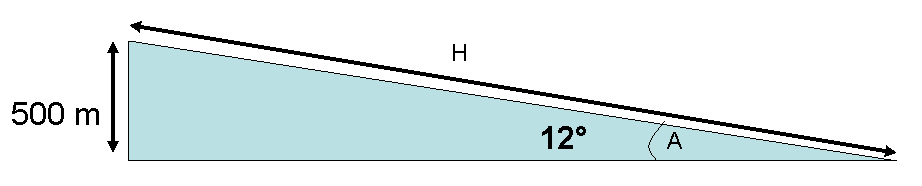You are walking up a 500. meter high hill. The trail has an incline of 12 degrees. How far will you walk to get to the top?
The first step is typically to make a sketch. However, this has been done already, so we will go to step 2, and determine which trig function to use.
We know angle A (12 degrees) and the length of the opposite side (500. m). We want to know the length of the hypotenuse (H), so we will use the the formula for the sine: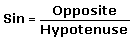Substituting in the appropriate values,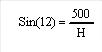Step 3 is to rearrange the equation so what you know is on one side and what you don't know is on the other, then substitute in the known values.
Finally, we will use a calculator to determine the value of the trigonometric function (sine in this case) and solve for the unknown value.
Using a calculator,
sin(12)=0.21 so,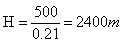The trail up the hill is 2400 m long.

### Depth to a bed of coal

You note that a bed coal is tilted at 12 degrees and comes to the surface 6 kilometers from your property. How deep will you have to dig to get to the coal bed on your property?
It may help to draw a picture of this. Try it yourself and then see if it looks like the one hidden below
Here we have an angle, 12 degrees, and know the adjacent side (6 km) and we want to know the length of the opposite side (O). The formula that will help us is the tangent:Substituting in the appropriate values,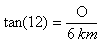Rearranging to isolate O,
O=tan(12)*6km
Using a calculator, the value of tan(12) is 0.213. So
O= 0.213*6km
So the opposite side of the triangle, the depth of the coal bed, is 1.275 km, or 1275 meters.

###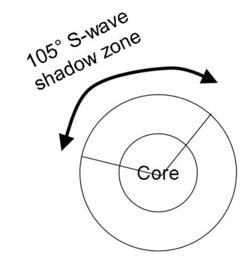Calculating radius of the outer core (seismology)

The S wave shadow zone is caused by the outer core not transmitting S waves. It crosses an arc of 105 degrees on the Earth (see the diagram on the left). Estimate the radius of the outer core. The radius of the entire Earth is 6370 km.

Again, it will be helpful to draw a diagram.
Here we have a right triangle with one 52.5 degree angle (half of 105 degrees) and a hypotenuse that is 6370 km long. We need to find the adjacent side, which is the approximate radius of the outer core, so we will use the cosine.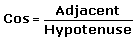Substituting in,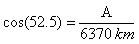Rearranging for A,
A= cos(52.5)*6370km
so
A= 3877 km
So the approximate radius of the core is 3877 km.
Note that this is approximate, because of the bending of the seismic waves as they reflect through the mantle. The actual radius is about 3500 km.

## Calculating an angle

The Colorado river drops from 3200 feet at Lake Mead to 500 feet elevation at Lee's Ferry, a river distance of 270 miles. What is the gradient in degrees?

Here we have two sides of a triangle and need an angle. The river distance is the hypotenuse and the vertical drop is the opposite angle. However, there is a catch. The measurements are not in the same units, and they must be to use our trigonometric formulas. So first, we'll convert the drop to miles (we could have converted the distance to feet - it doesn't matter.)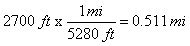(Note - if you need help with this step you can go to the unit conversions page )
Now we can use the formula for sine to calculate the angle xsubstituting in,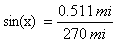so
sin(x)= 0.00189
to solve for x, the angle, take the inverse sine of each
sin-1(sin(x))= sin-1(0.00189)
since sin-1(sin(x))=x , our result is
x= 0.11 degrees
So the slope of the Colorado River is 0.11 degrees.

### Angle of Repose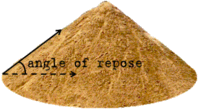The angle of repose is the steepest angle at which dry, unconsolidated sediment is stable. You create a conical pile of sand that is as steep as you can make it. The pile is 11 cm high and has a radius of 16 cm. What is the angle of repose of this sand?

Looking at the conical pile from the side, it is 2 right triangles put together. The height is the opposite side of the angle of repose and the radius of the pile is the adjacent side. So we have the information to fine the tangent of the angle of repose, which we can call x.so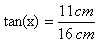tan(x)=.6875
From this we can solve for x, the angle of repose

to solve for x, we will need to take the inverse tangent of both sides of the equation
tan(x)=.6875
so
tan-1(tan(x))=tan-1(.6875)
since tan-1(tan(x))=x
x=34.5 degrees, the angle of repose for this sand.

### Plate tectonics and the angle of subduction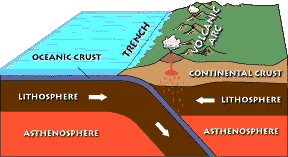Subducting oceanic slabs can plunge steeply or shallowly underneath the overriding plate. In modern subduction zones the location of earthquakes can show how steeply the subducting slab is plunging into the mantle, however in ancient subduction zones we cannot use this technique. Instead, we can use the observation that a volcanic arc typically occurs above the point at which the subducting plate reaches a depth of 100 km. If an ancient volcanic arc was 200 km from the trench that marks the initiation of subduction, what is the angle of subduction?

In this case one wants to find the angle of where the adjacent side (the distance to the volcanic arc) and the opposite side (the depth of the plate below the volcanic arc) are known, so we will need to use the tangent to find the angle of subduction.so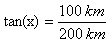Dividing, we find that
tan(x)=0.5
From this we can solve for x, the angle of subduction

to solve for x, we will need to take the inverse tangent of both sides of the equation
tan(x)=0.5
so
tan-1(tan(x))=tan-1(0.5)
since tan-1(tan(x))=x
x=26.6 degrees, the angle of subduction .

## Next Steps

If you feel comfortable with trigonometry, you can go on to the assessment.

Or you can go back to the trigonometry explanation page.